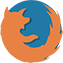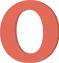## How can I calculate one point value?

You can use our Trader’s calculator to make the calculation. You can also calculate one point value using the following formula: OnePointValue = (Contract × (Price + OnePoint)) - (Contract × Price), where: • OnePointValue — one point value in the quote currency; • Contract — contract size in the base currency; • Price — price of the currency pair; • OnePoint — price tick (one point). Example. Calculation of one point value for GBPCHF on a USD account. • Lot – 1.25. • Trading instrument (currency pair) – GBPCHF. • GBPCHF rate – 1.47125. • Contract – 125 000 GBP. • USDCHF rate – 0.94950. OnePointValue = (125 000 x (1.47125 + 0.00001)) – (125 000 x 1.47125) = 183 907.5 - 183 906.25 = 1.25 CHF To convert the amount in USD: OnePointValue = 1.25 CHF / 0.94950 = 1.31 USD.

## List of questions

### Callback

A manager will call you shortly.